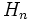Conjugacy-closed sub-APS

A sub-APS$H$ of an APS of groups$(G,\Phi)$ is termed conjugacy-closed if for every$n$,$H_n$ is a conjugacy-closed subgroup of$G_n$: in other words, whenever two elements of$H_n$ are conjugate in$G_n$, they are also conjugate in$H_n$.